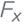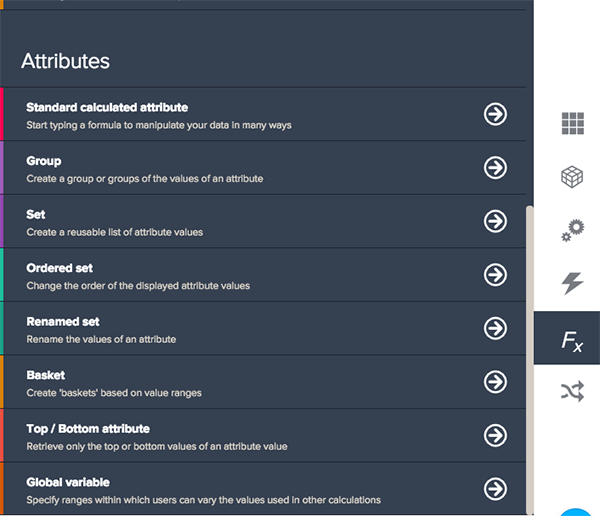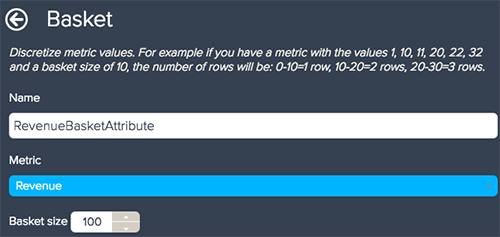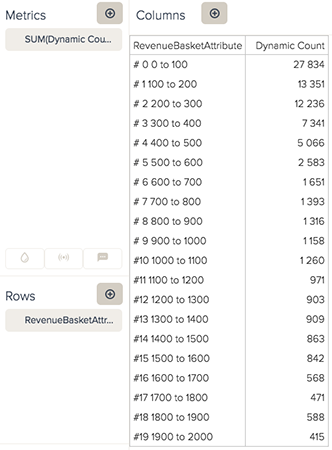# Dividing results into numeric ranges

Follow

Basket attributes divide your metrics into ranges, so you can see a distribution of your results. For example, if you have a list of sales, and want to see how many sales fall into a particular value bracket, you can use a basket attribute.

Note: Basket attributes are available for only in-memory data sources, such as Excel and CSV files.

Baskets are often used with a dynamic count or any other count you set up. A count aggregator enables you to see the number of results in each range easily.

To create a new basket attribute

1. Click the Calculation engine icon () on the right panel.
2. Select the Basket option underneath Attributes.3. Enter a name for your attribute.
4. Select a metric from the Metric drop-down list. The calculation will divide the selected metric into your basket's ranges.
5. Enter a basket range by typing a value or using the arrows in the Basket size box. Basket attributes are set up for equal repeating values, such as 10 to 100 ranges. If you want to create non-standard ranges (such as 1-10, 11-100, 101-1,000), you will have to create a standard calculated attribute.0 out of 0 found this helpful
Have more questions? Submit a request

•Jessica Marasco

Hi Nicholas,

This functionality is only available for in-memory data sources. Sorry for the confusion! I've made this more obvious in the doc.

Alternatively you can use a standard calculated attribute with nested IF THEN ELSE statements

IF ((SUM(Amount)>=0) AND (SUM(Amount)<10)) THEN
"0-10"
ELSEIF
((SUM(Amount)>=10) AND (SUM(Amount)<20))
THEN
"10-20"
ELSEIF
((SUM(Amount)>=20) AND (SUM(Amount)<30))
THEN "20 to 30"
ELSE
"not"
ENDIF
ENDIF
ENDIF

Please feel reach out to our Support team with any further questions. Thank you!

Edited by Jessica Marasco
• Was this functionality removed?